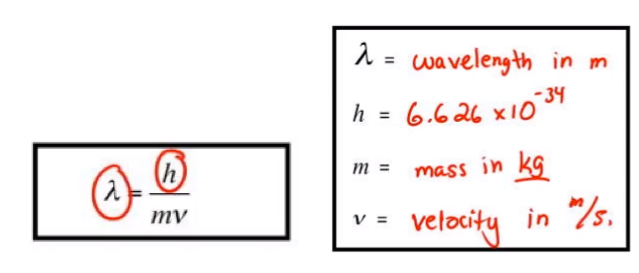# Problem: Compare the wavelengths of an electron (mass = 9.11 x 10 −31 kg) and a proton (mass = 1.67 x 10−27 kg), each having a speed of 3.4 x 10 6 m/s.

###### FREE Expert Solution

Use the de Broglie wavelength equation where:Plugging in the values of electron and proton:

87% (255 ratings)###### Problem Details

Compare the wavelengths of an electron (mass = 9.11 x 10 −31 kg) and a proton (mass = 1.67 x 10−27 kg), each having a speed of 3.4 x 10 6 m/s.